# 10道经典Java入门案例——保姆级教程，新手自学必备（建议收藏）

1年前   阅读数 162 0

🌲本文收录于专栏《技术专家修炼》

📚《源码中的设计模式》——理论和实战的完美结合

📚《leetcode 300题》——每天一道算法题，进大厂必备

📚《从实战学python》——Python的爬虫，自动化，AI等实战应用（代码开源）

Java入门练习100例1-10源码 提取码: uqqe

# 01.控制台打印——人生若只如初见，环境变量配一遍

## 知识点

• 配置环境变量
• 输出语句## 解题思路

### 1.环境搭建

“工欲善其事必先利其器”，即使输出语句很简单，没有运行环境，也是巧妇难为无米之炊。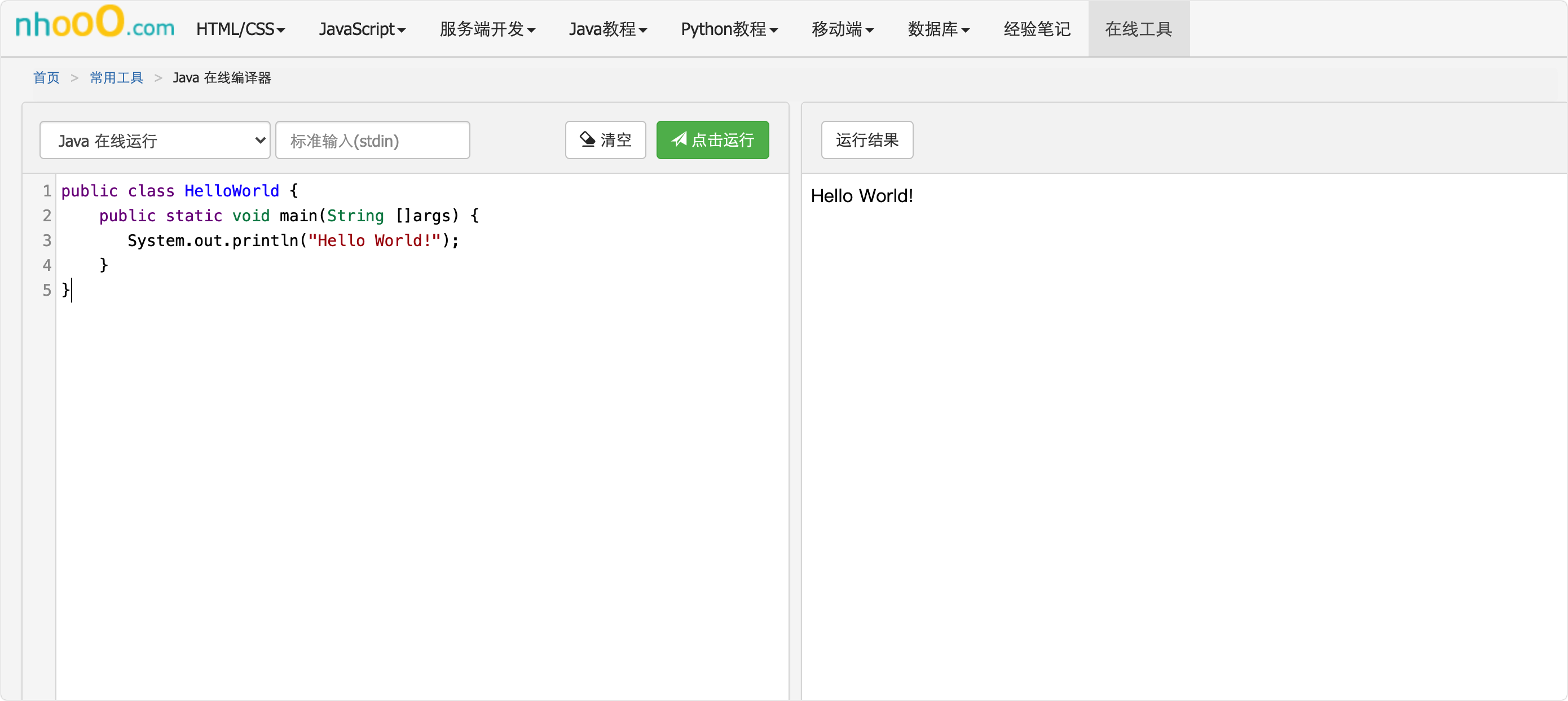Windows配置

Windows平台是使用者最多，配置起来最麻烦的平台，为了不被劝退，教大家最简单的方法。

1.安装JDK

2.打开环境变量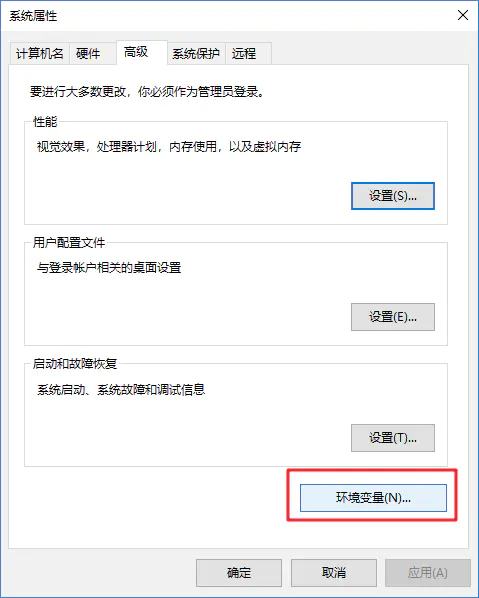3.新建JAVA_HOME

Mac配置

### 3.输出语句

`System.out.println("Hello Java!");`是Java语言的输出语句，相比于python的`print()`复杂了不少，那Java的输出语句到底是怎么实现的？

`System``java.long`包的类，在创建类文件时就被加载，不需要引入。被`final`修饰，不能被实例化。

``````public final class System {

// ……
}
``````

`out``System`类下的静态变量，对应`PrintStream`类，同样的还有`in``err`变量。

``````public final static PrintStream out = null;
``````

`println``PrintStream`类的方法，就是在这里实现IO流的输出。

``````    public void println(Object x) {

String s = String.valueOf(x);
synchronized (this) {

print(s);
newLine();
}
}
``````

## 代码实现

``````/** * https://www.nhooo.com/tool/java/ * 在控制台打印"hello java" */
public class question_01 {

public static void main(String[] args) {

System.out.println("hello java");
}
}
``````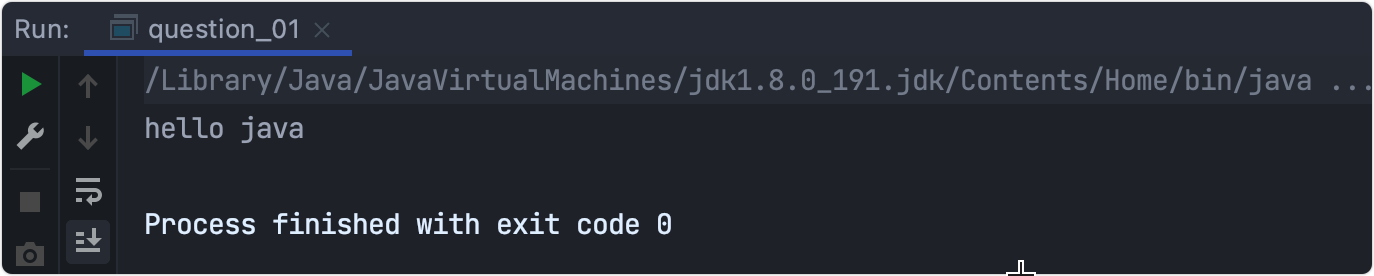# 02.键盘输入——三种方式

• 键盘输入
• 数学运算函数
• 类型转换

## 解题思路

### 1.键盘输入

• System.in

该方法能获取从键盘输入的字符，但只能针对一个字符的获取，获取的只是`char`类型的。如果想获得`int`,`float`等类型的输入，比较麻烦。具体见代码。

可以获取键盘输入的字符串，如果要获取的是`int`,`float`等类型的仍然需要转换。具体见代码。

• Scanner

可以获取键盘输入的字符串，有现成的获取`int`,`float`等类型数据，非常方便，被广泛使用。具体见代码。

### 2.数学运算函数

Java 的 `Math`类包含了用于执行基本数学运算的属性和方法，如初等指数、对数、平方根和三角函数。

Math 的方法都被定义为`static`形式，通过`Math`类可以在主函数中直接调用。

• `abs()` 返回参数的绝对值。
• `min()`返回两个参数中的最小值。
• `max()`返回两个参数中的最大值。
• `pow()`返回第一个参数的第二个参数次方。
• `sqrt()`求参数的算术平方根。
• `random()`返回一个随机数。
• `Math.PI`圆周率

### 3.类型转换

Java支持显示和隐式转换，在实际应用中要善于使用包装类的`parseXXX()``valueOf()`方法。

``````char ch = '9';
int i=ch-'0'
System.out.println(i);
``````

## 代码实现

``````import java.io.BufferedReader;
import java.io.IOException;
import java.util.Scanner;

/** * 从键盘输入一个数字，打印出该数字的绝对值。 */
public class question_02 {

public static void main(String[] args) throws IOException {

question_02 question02 = new question_02();
question02.method1();
// question02.method2();
// question02.method3();
}
//System.in
public void method1() throws IOException {

System.out.println("System.in --> "+Math.abs(i-'0'));
}
public void method2() throws IOException {

//读取一行，抛出异常
}
//Scanner
public void method3(){

Scanner sc = new Scanner(System.in);
//读取int
int i = sc.nextInt();
//String s = sc.nextLine(); 读取字符串型输入
//float f = sc.nextFloat(); 读取float型输入
System.out.println("Scanner --> "+Math.abs(i));
}
}
``````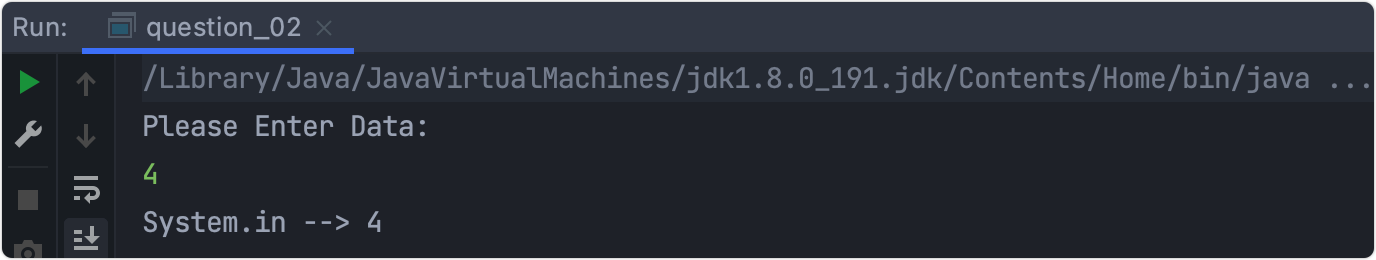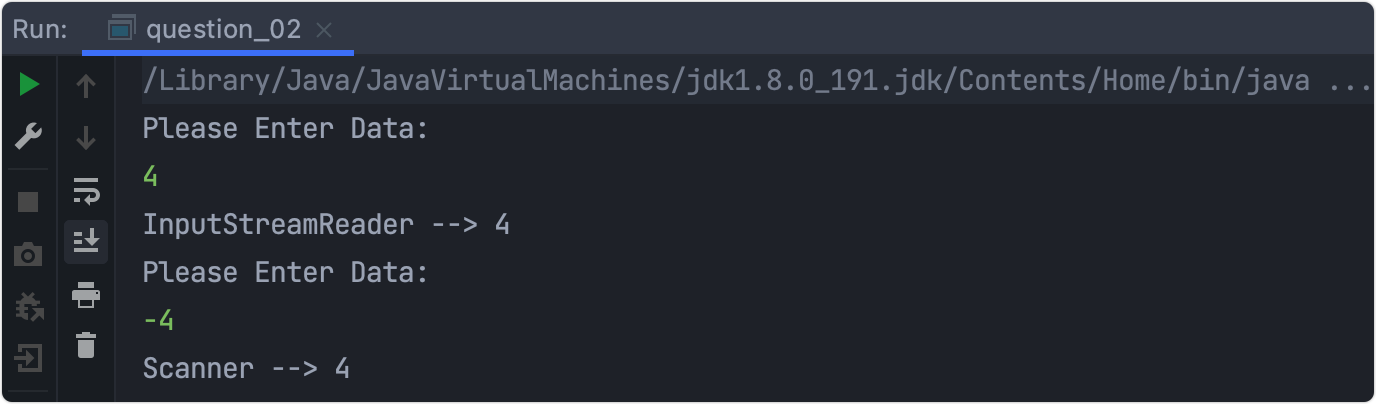# 03.短路与和逻辑与的区别——老实人和机灵鬼

## 题目描述

``````/** * 短路与和逻辑与 */
public class question_03 {

public static void main(String[] args){

int i=5;
boolean e = i > 6 & i++ > 7;
System.out.println(e);
System.out.println(i);
e = i > 6 && i++ > 7;
System.out.println(e);
System.out.println(i);
}
}
``````

• 关系运算符
• 自增运算

## 解题思路

• 逻辑与(&)和短路与(&&)在运算上对条件的结果判断不会产生影响,但会对条件本身的运算有影响。

• 逻辑与(&)在运算时会连续运算所有需要判断的命令.但短路与当遇到`false`时就停止运算。

## 代码实现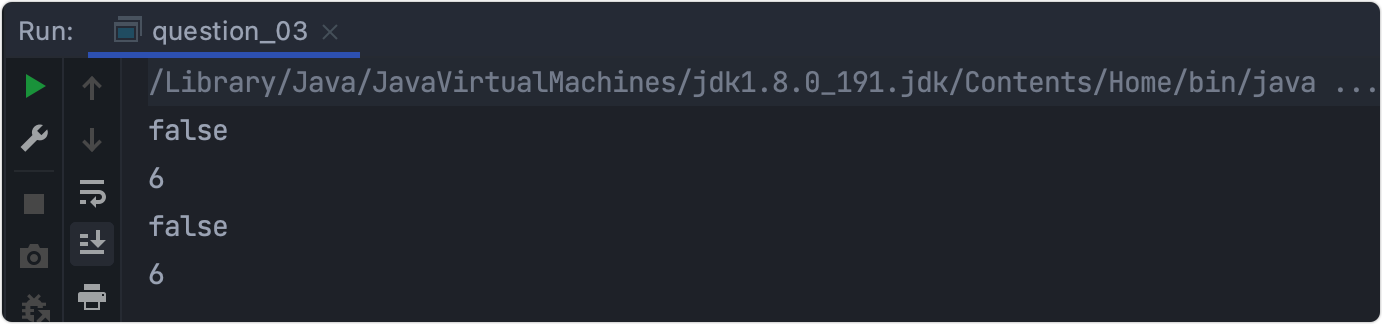# 04.三数排序——两数交换高级算法

## 知识点

• `if`判断
• 两数交换

## 解题思路

### 1.if判断

`if`作为一种分支结构，用来根据判断条件的不同做不同的后续处理。

### 2.两数交换

``````int t=a;
a=b;
b=t;
``````

## 代码实现

``````/** * 用if语句实现a、b、c的值按从小到大排序 */
public class question_04 {

public static void main(String args[]){

int a=9,b=5,c=7,t;
if(a>b) {

t=a; a=b; b=t;
}
if(a>c) {

t=a; a=c; c=t;
}
if(b>c) {

t=b; b=c; c=t;
}
System.out.println("a="+a+",b="+b+",c="+c);
}
}
``````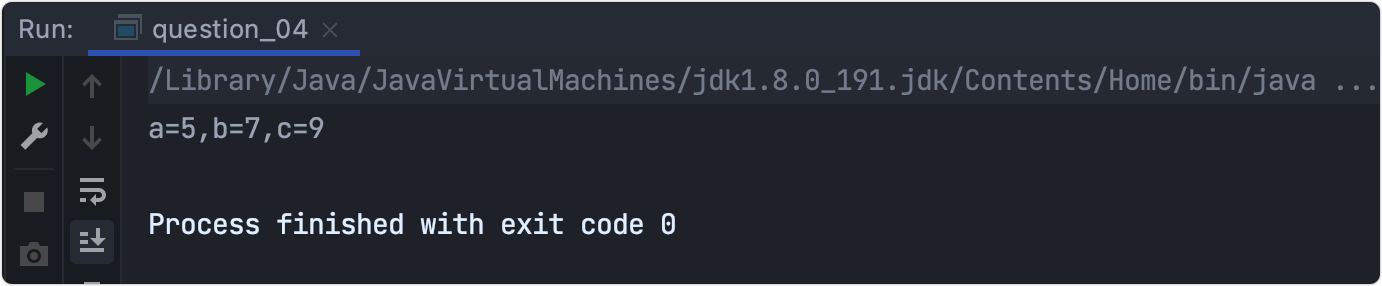## 总结

``````a=a*b;
b=a/b; //等价于 a*b/b=a,即将a的值赋给了b
a=a/b; //等价于 a*b/a=b,即将b的值赋给了a
``````

# 05.计算 5+ 55 + 555 + … 的前10项的和——for循环

• for循环
• 简单数学

## 解题思路

### 2.找规律

⚠️注意一点：最终的结果可能会超出`int`的范围，需要使用`long`

## 代码实现

``````/** *计算 5+ 55 + 555 + ... 的前10项的和 */
public class question_05 {

public static void main(String args[]){

//定义变量
long sum=0,a=5,item=a;
//初值1，步长1，终值10
for(int i=1;i<=10;i++) {

//追加到总和
sum=sum+item;
//确定下一个加数
item=item*10+a;
}
System.out.println(sum);
}
}
``````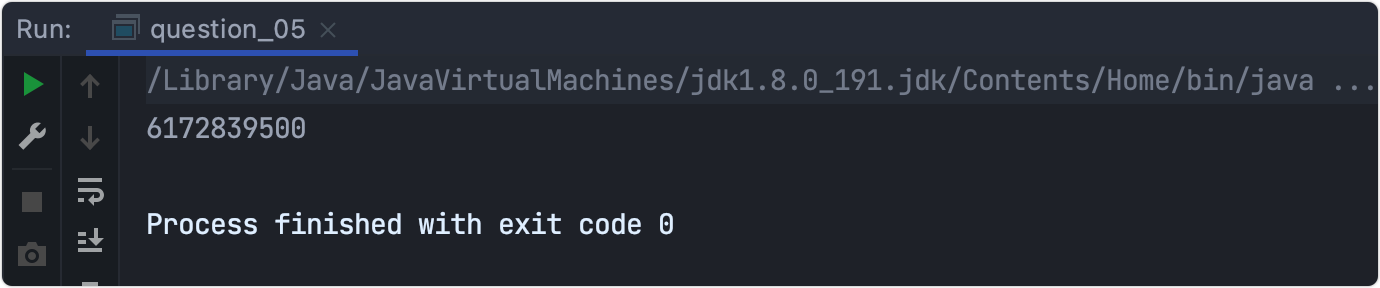# 06.计算 1 + 1/2! + 1/3! + 1/4! + … + 1/20! 的值——初窥动态规划

• 循环结构
• 阶乘的计算
• 初窥动态规划

## 解题思路

### 2.阶乘的计算

`n!`是为阶乘，等于`1*2*3*4...(n-1)*n`

## 代码实现

``````/** * 计算 1 + 1/2! + 1/3! + 1/4! + + 1/20! 的值 */
public class question_06 {

public static void main(String args[]) {

double sum=0,a=1;
int i=1;
while(i<=20) {

sum=sum+a;
i=i+1;
//关键点，动态规划思想
a=a*(1.0/i);
}
System.out.println("sum="+sum);
}
}
``````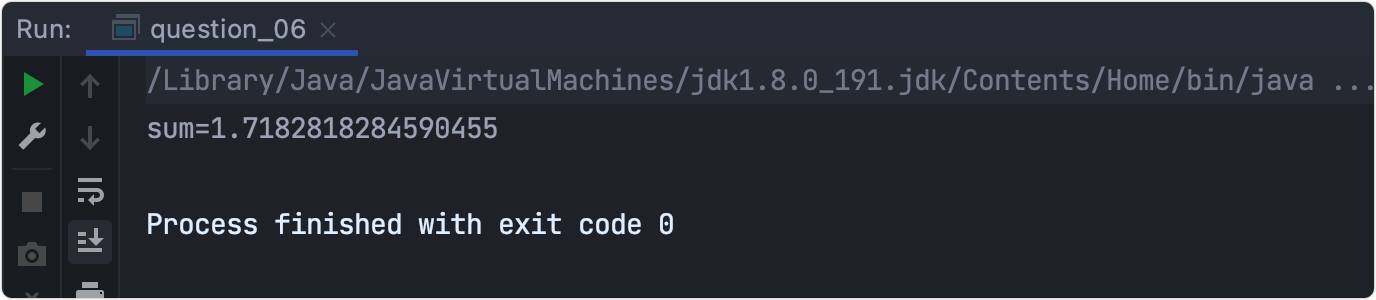# 07.各数字的和——取余运算

• 除法运算
• 取余运算

## 解题思路

``````int a=34;
//整除运算，拿到3
int b=34/10;
//返回余数4
int c=34%10;
``````

## 代码实现

``````/** * 计算给定整数12345的各数字的和。 */
public class question_07 {

public static void main(String args[]) {

int y = 12345;
int r = 0 ;
int sum = 0;
while(y!=0) {

r = y % 10;
sum += r;
y = y / 10;
}
System.out.println("y = " + sum);
}
}
``````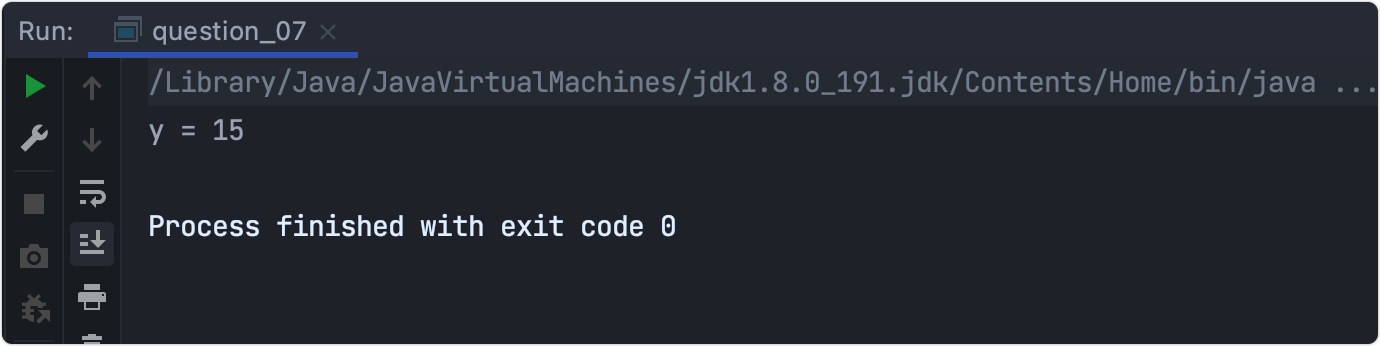# 08.素数和——break和continue

## 知识点

• 素数的定义
• `break``continue`
• 开方运算

## 解题思路

### 2.break和continue

`break`：终止所有循环，直接跳出。

`continue`：终止本次循环，继续执行下一次循环。

### 3.开方运算

`Math.sqrt()`

## 代码实现

``````/** * 求500以内的素数和 */
public class question_08 {

public static void main(String[] args) {

int sum=0,i,j;
for(j=2;j<=500;j++) {

for( i=2;i<=j/2;i++) {

if(j%i==0)
break; //说明除去1和本身有其他除数，不是素数，也就没有继续循环的必要
}
if(i>j/2) {
//i>j/2说明，break没有被执行到，即除去1和本身无其他除数，是素数
sum+=j;
}
}
System.out.println(sum);
}
}

``````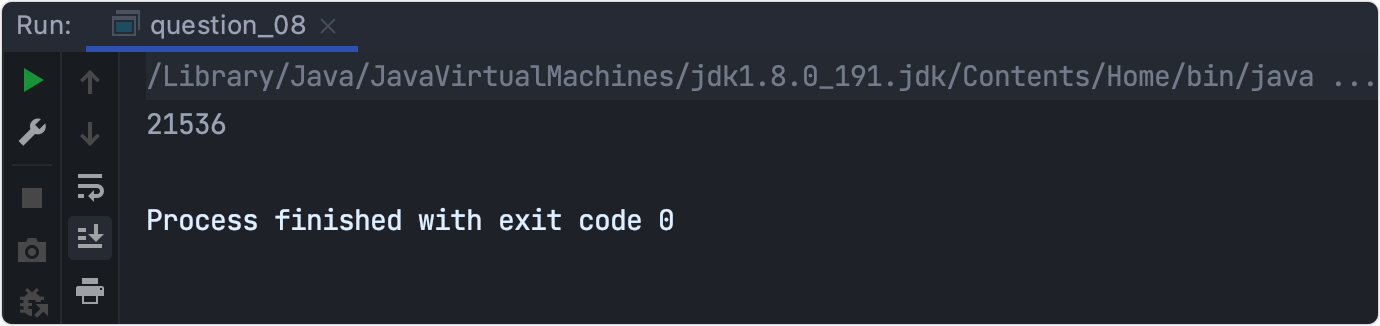# 09.数组中的最小值——一维数组

• 一维数组
• 排序

## 解题思路

### 1.什么是数组

``````int data[] = new int;
// 数组的长度为3，超过会报下标越界异常，且下标从0开始
``````

``````data = 10; // 第一个元素
data = 20; // 第二个元素
data = 30; // 第三个元素
``````

``````for(int x = 0; x < data.length; x++) {

System.out.println(data[x]); //通过循环控制索引
}
``````

## 代码实现

``````/** * 输出一维整型数组中的值最小的那个元素及其下标。 */
public class question_09 {

public static void main(String args[]) {

int a[] = {
12, 24, 6, 37, 3, 22, 64 };
int min = 0;
for (int i = 1; i < a.length; i++) {

if (a[min] > a[i]) {

min = i;
}
}
System.out.println("a[" + min + "] = " + a[min]);
}
}
``````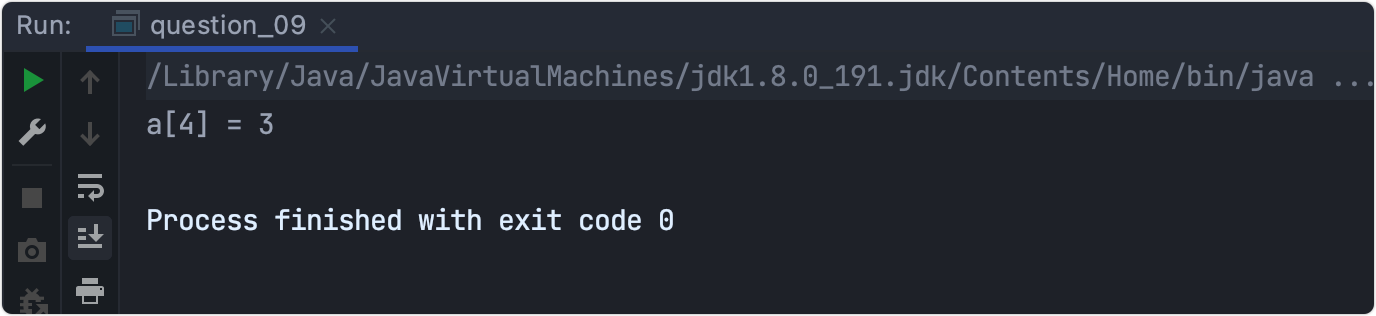# 10.各行元素之和——二维数组

• 二维数组
• 双重循环

## 解题思路

### 1.二维数组

``````int brr [] []=new int[]; //二维数组中行可以省略，至少写出列
``````

``````brr=1; //下标同样从0开始，可以指定位置赋值，也可以整体赋值。
brr={

{
1,2,3},
{
5,6,7},
{
9,10,11}
};
``````

``````//遍历行
for(int i=0;i<3;i++){

//遍历列
for(int j=0;j<3;j++){

System.out.println(brr[i][j]);
}
}
``````

## 代码实现

``````/** *计算二维数组中各行元素之和并查找其值最大的那个行。 */
public class question_10 {

public static void main(String args[]) {

int myTable[][] = {

{
22, 34, 45, 11, 33, 5, 92},
{
17, 9, 27, 31, 46, 54, 88},
{
98, 81, 64, 62, 15, 14, 23},
{
54, 43, 55, 1, 22, 9, 33}
};
int sum, max, maxRow=0;
max = 0;
for (int row=0; row<4; row++) {

sum = 0;
for (int col=0; col<7; col++)
sum += myTable[row][col];
if (sum > max) {

max = sum;
maxRow = row;
}
}
System.out.println("Row: " + maxRow + " value: " + max);
}
}

``````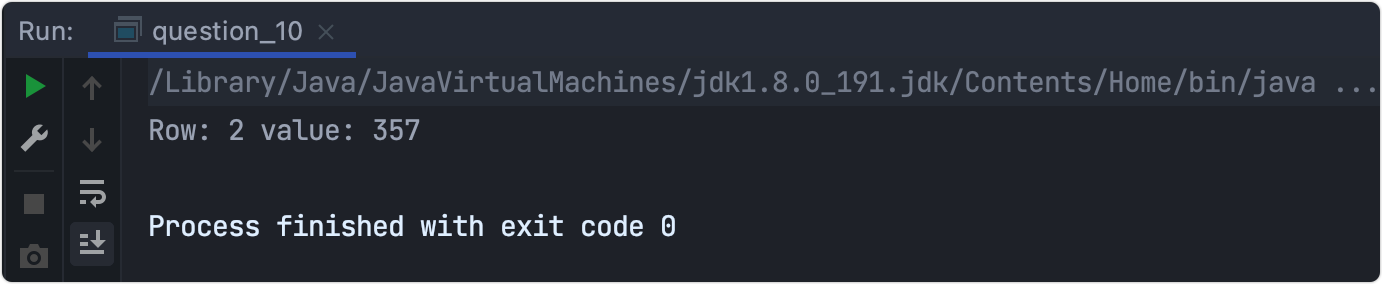## 扩展总结

11-100例正在来的路上。

1.5GJava学习资料点击下方👇卡片 关注后回复 资料

我有话说: